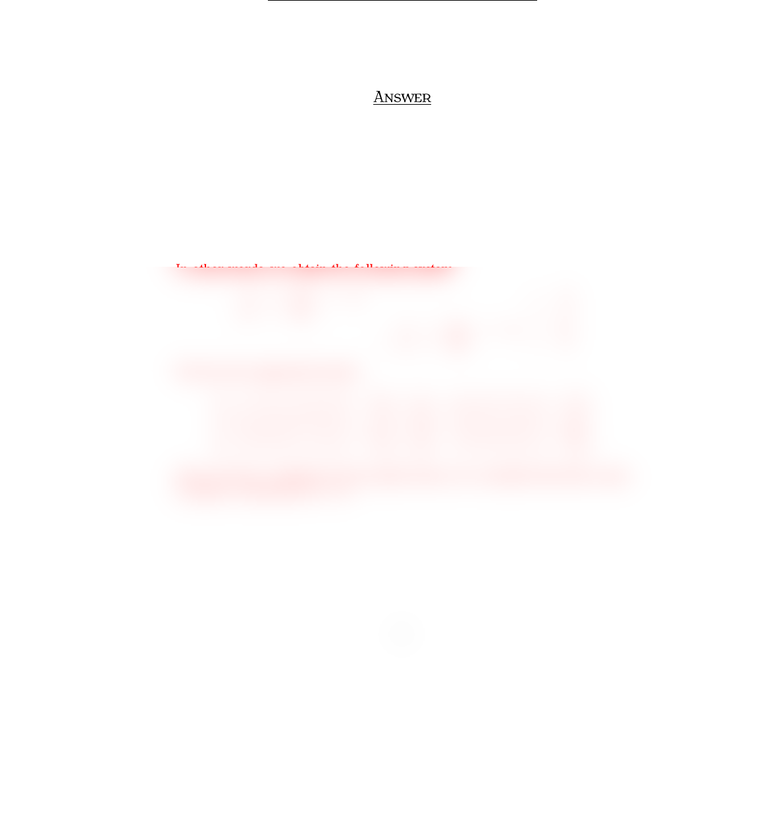# Quiz6mt210Sample2Ans

49 views1 pagesMT Q  S 
İ S. Y
M , 
Surname, Name:
Q . §. M O
Let A=
11
2 2
1 0
and B=[3 1
4 4 ]. Is there a matrix Csuch that
CA =B?
A
Let us say C=[x1x2x3
x4x5x6]so that CA =B. Then we get
[x1x2x3
x4x5x6]
11
2 2
1 0
=[3 1
4 4 ]
[x1+ 2x2+x3x1+ 2x2
x4+ 2x5+x6x4+ 2x5]=[3 1
4 4 ].
In other words, we obtain the following system
x1+ 2x2+x3= 3
x1+ 2x2= 1
x4+ 2x5+x6=4
x4+ 2x5= 4
We have the augmented matrix
121000 3
120000 1
0001214
000120 4
1200001
0 00120 4
0 000018
0 00000 0
Since we have a solution for the system above, we conclude that there exists
a matrix Csuch that CA =B.
1
Unlock document

This preview shows half of the first page of the document.
Unlock all 1 pages and 3 million more documents.